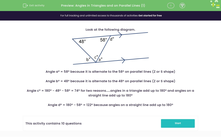# Calculate Angles in Triangles and on Parallel Lines

In this worksheet, students will use angle rules to work out the missing angles in triangles and parallel lines.Key stage:  KS 3

Curriculum topic:   Geometry and Measures

Curriculum subtopic:   Understand the Relationship Between Parallel Lines and Angles

Popular topics:   Angles worksheets

Difficulty level:#### Worksheet Overview

Look at the following diagram.

We can use our knowledge of angle rules to work out all the missing angles.Angle aº = 58º because it is alternate to the 58º on parallel lines (Z or S shape)

Angle bº = 48º because it is alternate to the 48º on parallel lines (Z or S shape)

Angle cº = 180º - 48º - 58º = 74º for two reasons.....angles in a triangle add up to 180º and angles on a straight line add up to 180º

Angle dº = 180º - 58º = 122º because angles on a straight line add up to 180º

Let's have a go at some questions now.

You can look back to this page for a reminder of the rules by clicking on the pink button at the side of the activity.

### What is EdPlace?

We're your National Curriculum aligned online education content provider helping each child succeed in English, maths and science from year 1 to GCSE. With an EdPlace account you’ll be able to track and measure progress, helping each child achieve their best. We build confidence and attainment by personalising each child’s learning at a level that suits them.

Get started